﻿ 鲁棒的正则化编码随机遮挡表情识别
«上一篇文章快速检索 高级检索

 智能系统学报2018, Vol. 13Issue (2): 261-268  DOI: 10.11992/tis.2016090020

### 引用本文LIU Shuaishi, GUO Wenyan, ZHANG Yan, et al. Recognition of facial expression in case of random shielding based on robust regularized coding[J]. CAAI Transactions on Intelligent Systems, 2018, 13(2): 261-268. DOI: 10.11992/tis.201609002.### 文章历史

Recognition of facial expression in case of random shielding based on robust regularized coding
LIU Shuaishi, GUO Wenyan, ZHANG Yan, CHENG Xi
College of Electrical and Electronic Engineering, Changchun University of Technology, Changchun 130000, China
Abstract: In order to improve facial expression recognition rate under the random shielding, a new face representation model was proposed: robust regularized coding. Regularized regression coefficients are used for carrying out robust regression for the given signals. Firstly, in order to reduce the influence of shielding on facial expression identification system, all pixels of the expression image to be identified will be assigned with different weights; then, because the occluded pixels should have lower weight values, hence, successive iteration is applied until the weight converges to the set weight threshold; finally, the sparse representation of image to be tested can be calculated by using the optimal weight matrix, in addition, the classified results of the expression image to be tested are determined by the minimal residual that the training samples approximate to the test image. The proposed method achieved an ideal performance in Japanese JAFFE expression database and Cohn-Kanade database, in addition, the experimental results show that the method is robust for the recognition of the facial expression randomly shielded.
Key words: random shielding    regularized coding    automatic update of weight    recognition of facial expression

1 鲁棒的正则化编码

 ${\hat{ \alpha }} = \arg \min {\left\| {{\alpha }} \right\|_1}{{s.t}}.\left\| {{{y}} - {{T\alpha }}} \right\|_2^2 \leqslant \varepsilon$ (1)

1.1 鲁棒的正则化编码模型

 ${\hat{ \alpha }} = \arg \max \left\{ {\ln P\left( {{{y}}|\left. {{\alpha }} \right) + \ln P\left( {{\alpha }} \right)} \right.} \right\}$ (2)

 ${\hat{\alpha }} = \arg \max \left\{ {\prod\limits_{i = 1}^n {{f_\theta }} \left( {{{{y}}_{{i}}} - {{{r}}_{{i}}}{{\alpha }}} \right)} + \prod\limits_{j = 1}^m {{f_0}} \left( {{{{\alpha }}_{{j}}}} \right)\right\}$ (3)

$\,{\rho _\theta }\left( {{e}} \right) = - \ln {f_\theta }\left( {{e}} \right)$ $\,{\rho _0}\left( {{\alpha }} \right) = - \ln {f_0}\left( {{\alpha }} \right)$ 式(3)转成：

 ${\hat{ \alpha }} = \arg \min \left\{ {\sum\limits_{i = 1}^n {{\rho _\theta }\left( {{{{y}}_{{i}}} - {{{r}}_{{i}}}{{\alpha }}} \right) + \sum\limits_{j = 1}^m {{\rho _0}\left( {{{{\alpha }}_{{j}}}} \right)} } } \right\}$ (4)

 ${f_0}\left( {{{{\alpha }}_{{j}}}} \right) = \beta \exp \left\{ { - {{\left( {\left| {{{{\alpha }}_{{j}}}} \right|/{\sigma _\alpha }} \right)}^\beta }} \right\}/\left( {2{\sigma _\alpha }\Gamma \left( {1/\beta } \right)} \right)$ (5)

1) ${\rho _\theta }\left( {0} \right)$ ${\rho _\theta }\left( {{e}} \right)$ 的局部最小值；

2) 对称性： ${\rho _\theta }\left( {{{{e}}_{{i}}}} \right) = {\rho _\theta }\left( { - {{{e}}_{{i}}}} \right)$

3) 单调性：当 $\left| {{{{e}}_{{1}}}} \right| > \left| {{{{e}}_{{2}}}} \right|$ 时， ${\rho _\theta }\left( {{{{e}}_{{1}}}} \right) > {\rho _\theta }\left( {{{{e}}_{{2}}}} \right)$ 。不失一般性，令 ${\rho _\theta }\left( {0} \right) =0$

1.2 迭代权重优化鲁棒的正则化编码模型

 ${\tilde F_\theta }\left( {{e}} \right) = {F_\theta }\left( {{{{e}}_{{0}}}} \right) + {\left( {{{e}} - {{{e}}_{{0}}}} \right)^{ T}}{F'_\theta }\left( {{{{e}}_{{0}}}} \right) + {R_1}\left( {{e}} \right)$ (6)

${F_\theta }\left( {{e}} \right)$ ${{e}} = 0$ 取得最小值的同时，它的近似值 ${\tilde F_\theta }\left( {{e}} \right)$ ${{e}} = 0$ 也应取得最小值。令 ${F'_\theta }\left( 0 \right) = 0$ ，可以得到 ${{W}}$ 的对角元素如式(7)：

 ${{{W}}_{{{{i}},{{i}}}}} = {\rho '_\theta }\left( {{{{e}}_{{{{0}},{{i}}}}}} \right)/{{{e}}_{{{{0}},{{i}}}}}$ (7)

 ${\hat{ \alpha }} = \arg \min \left\{ {\frac{1}{2}\left\| {{{{W}}^{1/2}}\left( {{{y}} - {{T\alpha }}} \right)} \right\|_2^2 + \sum\limits_{j = 1}^m {{\rho _0}\left( {{{{\alpha }}_{{j}}}} \right)} } \right\}$ (8)

1.3 权重W

${{{W}}_{{{{i}},{{i}}}}}$ 表示分配给待测表情图像 ${{y}}$ 每个像素点 $i$ 的权值。从人的感官认识出发，被遮挡部分的像素点应该具有较低的权重，这样可以减少它们对编码过程的影响。由于完备字典是由非遮挡的人脸表情图像构成的，可以很好地表征人脸表情，然而遮挡部分像素造成的异常值将具有较大的编码残差，因此，这些具有较大的编码残差像素点应具有较小的权重。通过观察式(7)可以得到 ${{{W}}_{{{{i}},{{i}}}}}$ ${{{e}}_{{i}}}$ 成反比，与 ${\rho '_\theta }\left( {{{{e}}_{{i}}}} \right)$ 成正比。由于ρθ可微、对称、单调并且在原点取得最小值，可以假设 ${{{W}}_{{{{i}},{{i}}}}}$ 是连续且对称的，与 ${{{e}}_{{i}}}$ 成反比而且有界。不失一般性，令 ${{{W}}_{{{{i}},{{i}}}}} \in \left[ {0,1} \right]$ ，综合多方面的考虑，逻辑函数是权重函数的最好选择。本文选用与逻辑函数具有相似性质的SVM hinge loss函数作为权重函数。

 ${\left\| {{{{W}}^{\left( t \right)}} - {{{W}}^{\left( {t - 1} \right)}}} \right\|_2}/{\left\| {{{{W}}^{\left( {t - 1} \right)}}} \right\|_2} < \gamma$ (9)

 ${r_i}\left( {{y}} \right) = {\left\| {{{W}}_{{final}}^{1/2}\left( {{{y}} - {{T}}{{{\delta }}_{{i}}}\left( {{\hat{ \alpha }}} \right)} \right)} \right\|_2},i = 1,2, \cdots ,k$ (10)

 ${{identity}}\left( {{y}} \right) = \arg \mathop {\min }\limits_{i \in \left( {1, 2, \cdots ,k} \right)} \left( {{r_i}\left( {{y}} \right)} \right)$ (11)

 ${\hat{ \alpha }} = \arg \min \left\{ {\frac{1}{2}\left\| {{{P}}{{{W}}^{1/2}}\left( {{{y}} - {{T\alpha }}} \right)} \right\|_2^2 + \sum\limits_{j = 1}^m {{\rho _0}\left( {{{{\alpha }}_{{j}}}} \right)} } \right\}$ (12)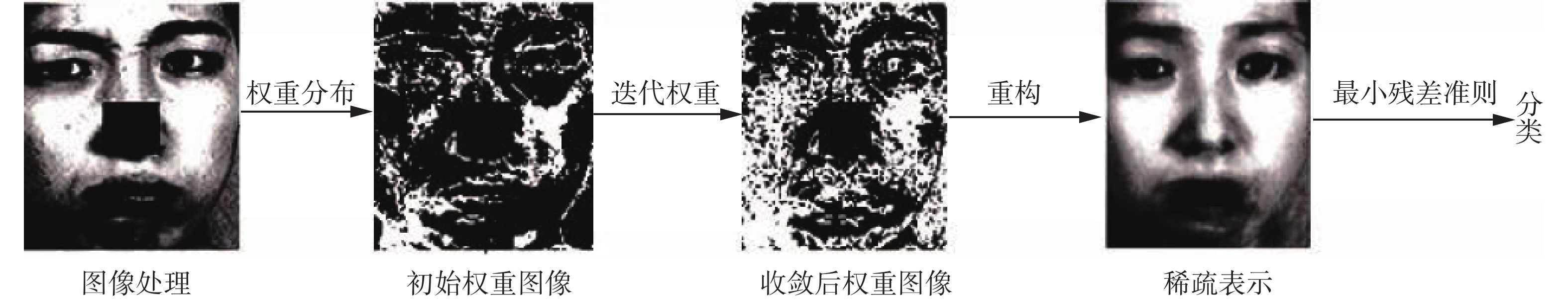Download: 图 1 本文方法的流程 Fig. 1 The structure of our method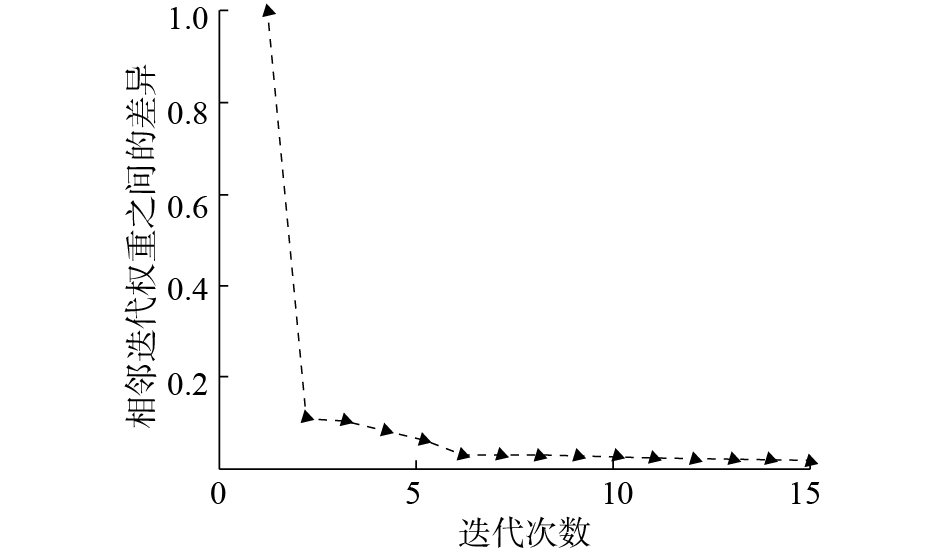Download: 图 2 权重收敛曲线 Fig. 2 The convergence curve of the weigh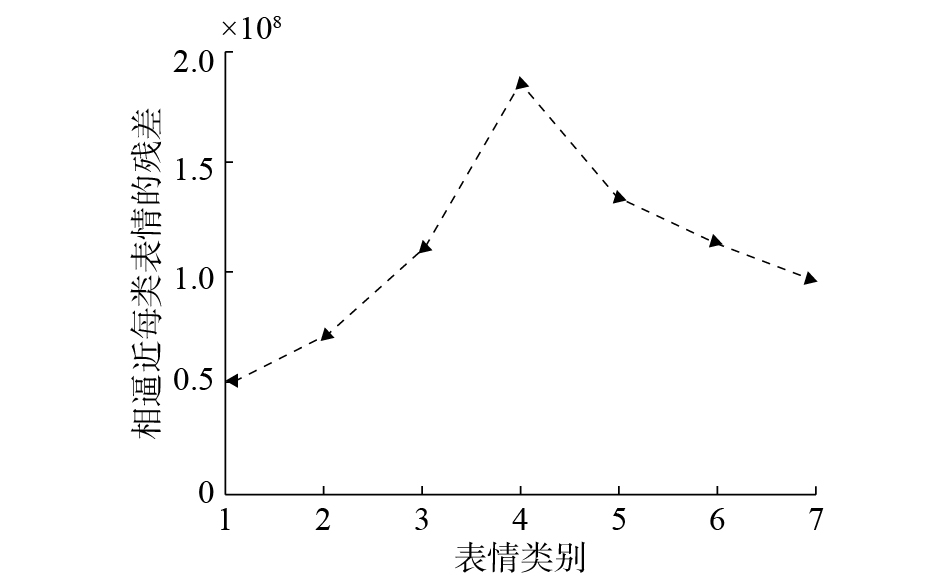Download: 图 3 不同类别训练图像逼近待测图像的残差图 Fig. 3 The residual of each training class approximates the test image
2 实验描述与结果分析

2.1 实验描述Download: 图 4 实验用的两数据库中的部分随机遮挡表情图像 Fig. 4 Some samples of occluded facial images in two databases

2.2 结果分析表 1 不同方法在JAFFE数据库上的识别率 Tab.1 The accuracies of different methods on JAFFE表 2 不同方法在Cohn-Kanade数据库上的识别率 Tab.2 The accuracies of different methods on Cohn-Kanade表 3 每种表情在JAFFE数据库不同遮挡级别的识别率 Tab.3 The accuracies of each expression on JAFFE in different levels of block occlusions表 4 每种表情在Cohn-Kanade数据库不同遮挡级别的识别率 Tab.4 The accuracies of each expression on Cohn-Kanade in different levels of block occlusions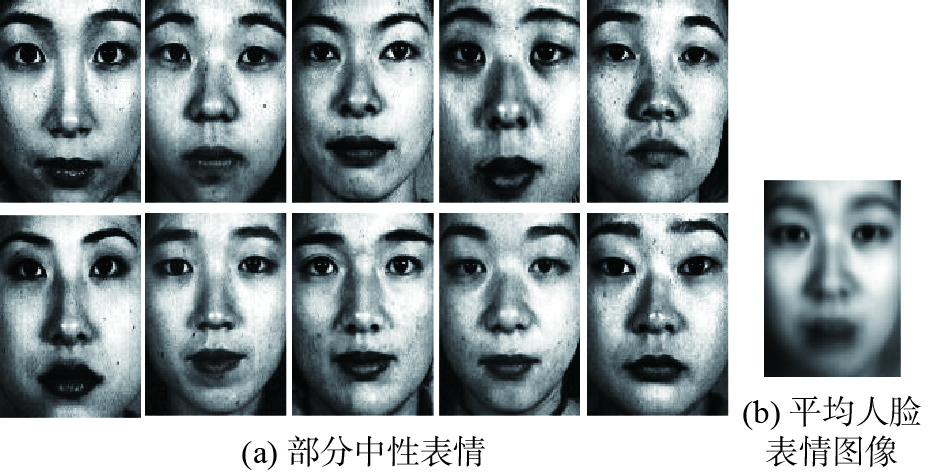Download: 图 5 JAFFE数据库 Fig. 5 JAFFE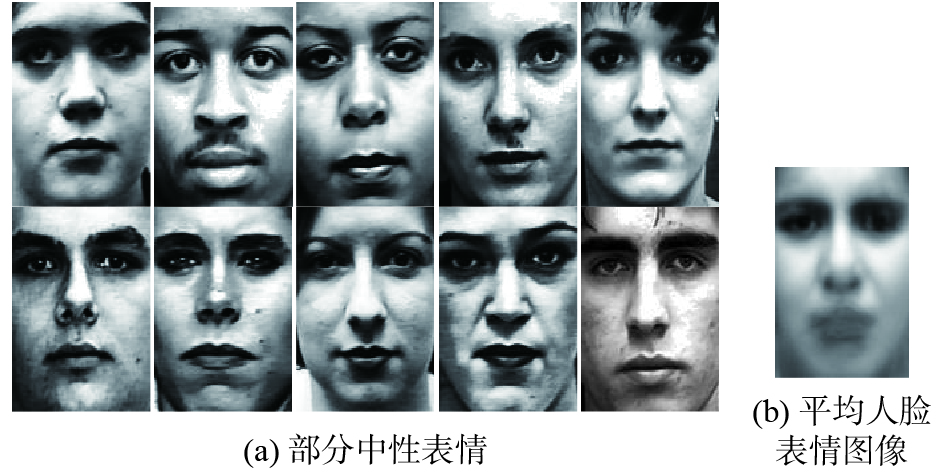Download: 图 6 Cohn-Kanade数据库 Fig. 6 Cohn-Kanade
3 结束语

  MERY D, BOWYER K. Face recognition via adaptive sparse representations of random patches[C]//IEEE International Workshop on Information Forensics and Security. London, UK, 2015: 13–18. (0)  WANG J, LU C, WANG M, et al. Robust face recognition via adaptive sparse representation[J]. IEEE transactions on cybernetics, 2014, 44(12): 2368. DOI:10.1109/TCYB.2014.2307067 (0)  赵军, 赵艳, 杨勇. 基于降维的堆积降噪自动编码机的表情识别方法[J]. 重庆邮电大学学报: 自然科学版, 2016, 28(6): 844-848. ZHAO Jun, ZHAO Yan, YANG Yong, et al. Facial expression recognition method based on stacked denoising auto-encoders and feature reduction[J]. Journal of Chongqing university of posts and telecommuncaitions: natual science edtion, 2016, 28(6): 844-848. (0)  KOTSIA I, PITAS I, ZAFEIRIOU S, et al. Novel multiclass classifiers based on the minimization of the within-class variance[J]. IEEE transactions on neural networks, 2009, 20(1): 14-34. DOI:10.1109/TNN.2008.2004376 (0)  TARRÉS F, RAMA A, TORRES L. A novel method for face recognition under partial occlusion or facial expression variations[C]//Proceedings of the 47th International Symposium ELMAR. Zadar, Croatia, 2005: 163–166. (0)  KOTSIA I, BUCIU I, PITAS I. An analysis of facial expression recognition under partial facial image occlusion[J]. Image and vision computing, 2008, 26(7): 1052-1067. DOI:10.1016/j.imavis.2007.11.004 (0)  ZHANG Ligang, TJONDRONEGORO D, CHANDRAN V. Toward a more robust facial expression recognition in occluded images using randomly sampled Gabor based templates[C]//Proceedings of 2011 IEEE International Conference on Multimedia and Expo. Barcelona, Spain, 2011: 1–6. (0)  王晓华, 李瑞静, 胡敏. 融合局部特征的面部遮挡表情识别[J]. 中国图象图形学报, 2016, 21(11): 1473-1482. WANG Xiaohua, LI Ruijing, HU Min, et al. Occluded facial expression recognition based on the fusion of local features[J]. Journal of image and graphics, 2016, 21(11): 1473-1482. DOI:10.11834/jig.20161107 (0)  WRIGHT J, YANG A Y, GANESH A, et al. Robust face recognition via sparse representation[J]. IEEE transactions on pattern analysis and machine intelligence, 2009, 31(2): 210-227. DOI:10.1109/TPAMI.2008.79 (0)  朱明旱, 李树涛, 叶华. 基于稀疏表示的遮挡人脸表情识别方法[J]. 模式识别与人工智能, 2014, 27(8): 708-712. ZHU Minghui, LI Shutao, YE hua. An occluded facial expression recognition method based on sparse representation[J]. Pattern recognition and artificial intelligence, 2014, 27(8): 708-712. (0)  WRIGHT J, YANG A Y, GANESH A, et al. Robust face recognition via sparse representation[J]. IEEE transactions on pattern analysis and machine intelligence, 2009, 31(2): 210-227. DOI:10.1109/TPAMI.2008.79 (0)  CAO J, ZHANG K, LUO M, et al. Extreme learning machine and adaptive sparse representation for image classification[J]. Neural networks the official journal of the international neural network society, 2016, 81(c): 91. (0)  ZHANG Jian, JIN Rong, YANG Yiming. Modified logistic regression: an approximation to SVM and its applications in large-scale text categorization[C]//Procee-dings of the Twentieth International Conference on Machine Learning. Washington, DC, USA, 2003: 888–895. (0)  LIU Shuaishi, ZHANG Yan, LIU Keping, et al. Facial expression recognition under partial occlusion based on Gabor multi-orientation features fusion and local Gabor binary pattern histogram sequence[C]//Proceedings of the 9th International Conference on Intelligent Information Hiding and Multimedia Signal Processing. Beijing, China, 2013: 218–222. (0)  LIU Licheng, CHEN Long, CHEN C L. Weighted joint sparse representation for removing mixed noise in image[J]. IEEE transactions on cybernetics includes computational approaches to the field of cybernetics, 2016: 1-12. (0)  YANG M, SONG T, LIU F, et al. Structured regularized robust coding for face recognition[J]. IEEE transactions on image processing a publication of the IEEE signal processing society, 2013, 22(5): 1753-1766. DOI:10.1109/TIP.2012.2235849 (0)  罗元, 吴彩明, 张毅. 基于PCA与SVM结合的面部表情识别的智能轮椅控制[J]. 计算机应用研究, 2012, 29(8): 3166-3168. LUO Yuan, WU Caiming, ZHANG Yi, et al. Facial expression recognition based on principal component analysis and support vector machine applied in intelligent wheelchair[J]. The research and application of computer, 2012, 29(8): 3166-3168. (0)